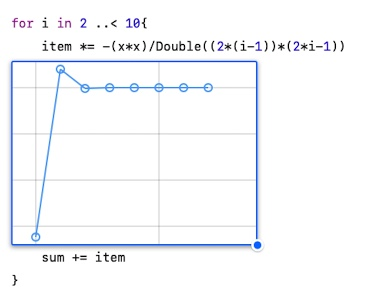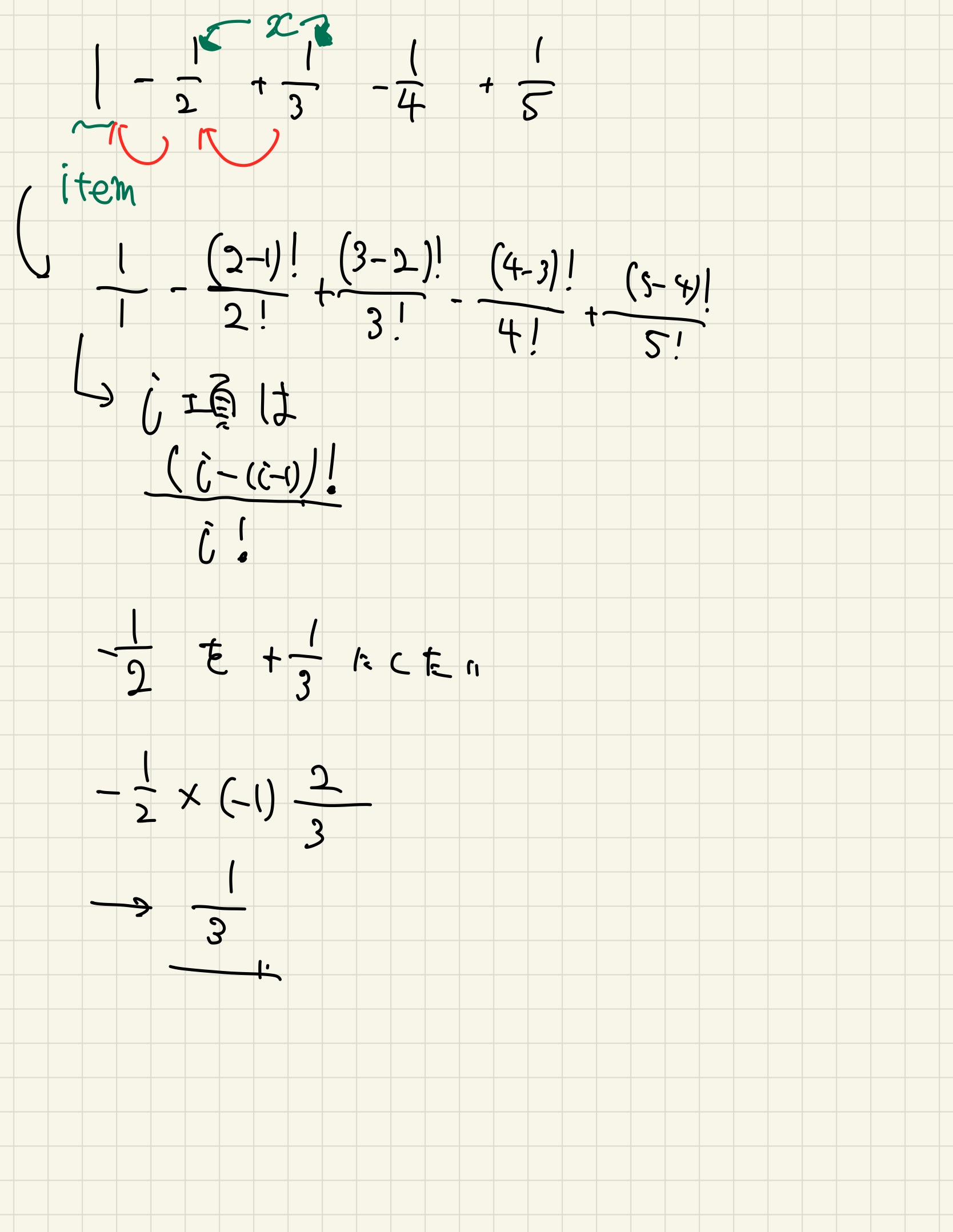# Find the approximate value of log (1 + x) in Swift

## Why Swift?

Swift does not need to build an environment, if you have a Mac or iPad, just install Playgrounds and use it be able to. In addition, Playgrounds has functions such as ** step execution ** and ** slow execution **, and you can execute for statements and while minutes step by step and check the change in the value when it is executed. .. Furthermore, if you use the ** viewer function **, the state of variable changes will be displayed as a graph without permission.## McLaughlin expansion of log (x + 1)

The McLaughlin expansion of $\ log (1 + x)$

\log(1+x) = x-\frac{x^2}{2}+\frac{x^3}{3}-\frac{x^4}{4}+⋯+\frac{(-1)^{n-1}x^n}{n}+⋯

It was expressed as. In this article, we will use programming to find the approximate value of $\ log (1 + x)$. (Language is Swift) ipad is fine.

## 1. Prepare variables

The McLaughlin expansion approximates the function $f (x)$, which can be differentiated many times, by the sum of the expansion formulas of the series of the power function of $x$. In this article, the value of $\ log (x + 1)$ is set as sum, and the value is gradually added to sum.

var sum = 0
print(sum)    //0
sum += 1
print(sum)    //1


You also have to prepare a constant to put the approximate $x$.

var sum = 0
let x = 1


In addition, prepare variables that are continuously updated as terms.

var item = x


The first term is the same as the constant, so enter x.

The final code looks like this:

var sum = 0.0  //Since int type and double type cannot be added,0.Write 0 to make it a double type.
let x = 1.0
var item = x


## 2. Code up to the second term with x = 1

I think it is important to start with a small value and try and error with a concrete value when coding. (Personal view). In this article as well, according to that idea, $x = 1$ up to the second term, that is,

\log (1+x)=x-\frac{x^2}{2}

Code up to.

var sum = 0.0  //Since int type and double type cannot be added,0.Write 0 to make it a double type.
let x = 1.0
var item = x

sum += 1.0
sum += -(x*x)/Double(2*1)


Finally, add a print statement to compare with the true value.

import Foundation
var sum = 0.0  //Since int type and double type cannot be added,0.Write 0 to make it a double type.
let x = 1.0
var item = x

sum += 1.0
sum += -(x*x)/Double(2*1)
print(sum)//Approximate value 0.5
print(log(2.0))//True value 0.6931471805599453


## 3. Make it easy to generalize

Earlier we set the second term to $-\ frac {x ^ 2} {2}$, but with this we have to increase $x$ and mess with the denominator as the number of terms increases. Add some ideas so that you can code short even if the number of terms increases.

import Foundation
var sum = 0.0  //Since int type and double type cannot be added,0.Write 0 to make it a double type.
var item = 1.0
let x = 1.0
sum += item

for i in 2 ..< 3{
item *= -(x*Double(i-1))/Double(i)
sum += item
}
print(sum)//Approximate value 0.5
print(log(2.0))//True value 0.6931471805599453


Observing the McLaughlin-expanded $\ log (x + 1)$, $x$ and $1 / n$ are multiplied each time the term grows, so express it in code.The for statement is used, but the sum value does not change because it is executed only once.

## 4. Increase the number of terms

It can be dealt with by increasing the number of terms by generalizing.

import Foundation
var sum = 0.0  //Since int type and double type cannot be added,0.Write 0 to make it a double type.
var item = 1.0
let x = 1.0
sum += item

for i in 2 ..< 10{
item *= -(x*Double(i-1))/Double(i)
sum += item
}
print(sum)//Approximate value 0.7456349206349205
print(log(2.0))//True value 0.6931471805599453


Even if the item 9 is added, there is a considerable difference from the true value. Since $sinx and cosx$ were almost the same in the sixth term, it can be seen that $\ log (x + 1)$ converges more slowly than $sin$ and $cos$.